# List of problems in mathematical notation

This page gives a list of problems in mathematical notation. The list focuses on problems with the symbolism of mathematics rather than other communication problems/conventions (such as using "if" to mean "iff" in definitions, not introducing variables, etc.).

Name Description How to avoid
Overloading/abuse of notation This happens when the same symbol is used for different purposes. For instance, parentheses are used to group expressions, for writing tuples, for function calls, in superscripts or subscripts with various meanings ($f^{(i)}$ for the$i$th derivative or the$i$th function in some sequence), and so forth. The equals sign is often used for equality both in the object language and the metalanguage in mathematical logic, and used as part of the expression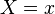$X=x$ to define an event in probability. 0 in linear algebra to mean the scalar 0 as well as the 0 vector of any dimension.
Type error
Failure of Leibniz law, "same object denotes different things" in probability, where$\Pr(X=x)$ is abbreviated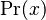$\Pr(x)$. Then if we take something like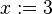$x:=3$, we would have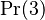$\Pr(3)$, but now we have lost the information about what random variable we are talking about.
"different objects denoted by the same thing" see e.g. here, where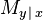$M_{y\,|\,x}$ can mean two different things on each side of an equation.
Omission of index Writing things like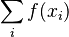$\sum_i f(x_i)$ (unclear what set the index ranges over, or the order in which the terms are added, which can sometimes matter) or even just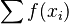$\sum f(x_i)$ (unclear which variable is the index).
Ambiguous order of operations e.g.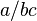$a/bc$
Undefined operations e.g. I often see in mathematical logic things like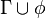$\Gamma \cup \phi$ to mean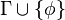$\Gamma \cup \{\phi\}$, where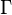$\Gamma$ is a set of formulas and$\phi$ is a formula.
strange reorderings based on context e.g. https://machinelearning.subwiki.org/wiki/User:IssaRice/Minus_notation_in_game_theory